# Is there a formula for these circles?

I have six 2x2 complex matrices from the group SL(2,C). Each line is a matrix: first row, second row.

$$\left( \begin{array}{cc} \left\{\frac{1}{\sqrt{1-k^2}},\frac{k}{\sqrt{1-k^2}}\right\} & \left\{\frac{k}{\sqrt{1-k^2}},\frac{1}{\sqrt{1-k^2}}\right\} \\ \left\{\frac{1}{\sqrt{1-k^2}},\frac{i k}{\sqrt{1-k^2}}\right\} & \left\{-\frac{i k}{\sqrt{1-k^2}},\frac{1}{\sqrt{1-k^2}}\right\} \\ \left\{\frac{k+1}{\sqrt{1-k^2}},0\right\} & \left\{0,\frac{1-k}{\sqrt{1-k^2}}\right\} \\ \left\{\frac{1-k}{\sqrt{1-k^2}},0\right\} & \left\{0,\frac{k+1}{\sqrt{1-k^2}}\right\} \\ \left\{\frac{1}{\sqrt{1-k^2}},-\frac{i k}{\sqrt{1-k^2}}\right\} & \left\{\frac{i k}{\sqrt{1-k^2}},\frac{1}{\sqrt{1-k^2}}\right\} \\ \left\{\frac{1}{\sqrt{1-k^2}},-\frac{k}{\sqrt{1-k^2}}\right\} & \left\{-\frac{k}{\sqrt{1-k^2}},\frac{1}{\sqrt{1-k^2}}\right\} \\ \end{array} \right)$$

Let us take $k=0.7$ and act with these matrices on the complex plane via linear fractional transformations (Mobius transformations):
$$z\mapsto\frac{az+b}{cz+d}$$.
Selecting an arbitrary starting point and applying consecutively randomly selecting matrices several millions of times ("Chaos Game") we create a pattern. Here is the pattern in the square $-8<x,y<8$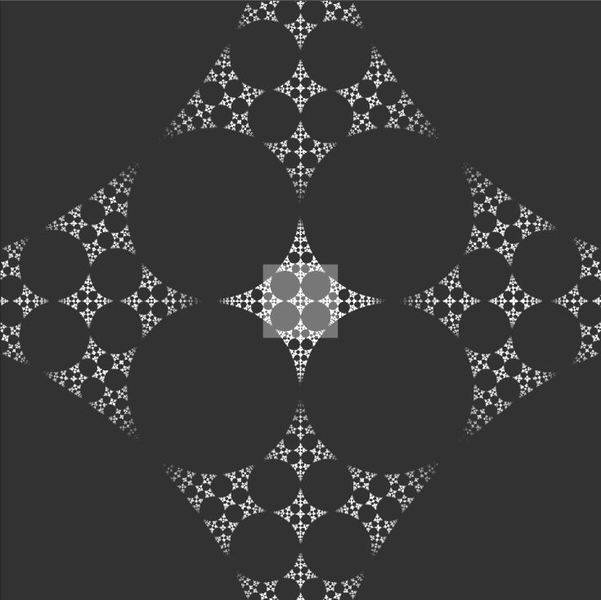The pattern seems to be self-similar. Here is the central square $-1<x,y<1$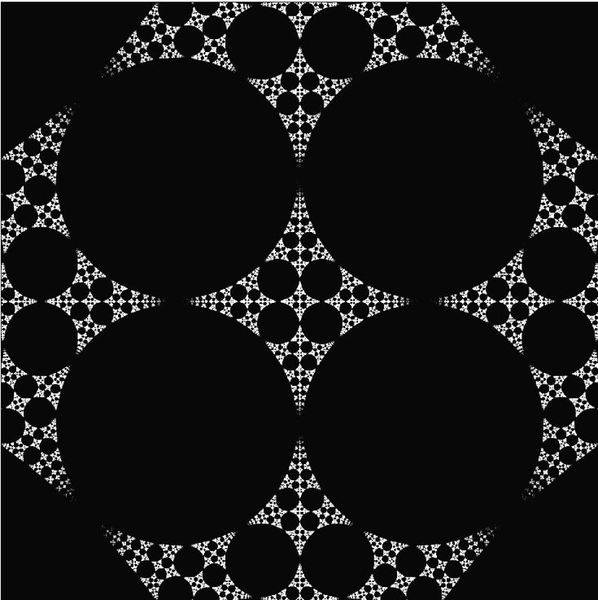Question: Is it possible, looking at the transformations to find a formula for the centers and the radii of all these circles? What kind of a formula would it be? A closed algebraic expression? An algorithm?

P.S. You can create this pattern yourself using Quantum Fractal Generator. Just choose Alpha=0.7, Chaos Game, Fractal Type: PlatonicOcta, Display Type Stereographic, XY LIMIT 8.0, Fractal Algorithm: Chaos Game, Color Scheme: Grayscale, 10 mln iterations.
P.S2: Your computer will crash if you try to implement the transformations as described in my post. The trick is to do it on the Riemann sphere rather than on the complex plane.

Last edited:

Update

After asking the above question I started playing with numbers. I think I have the formula for the radius of the central white circle: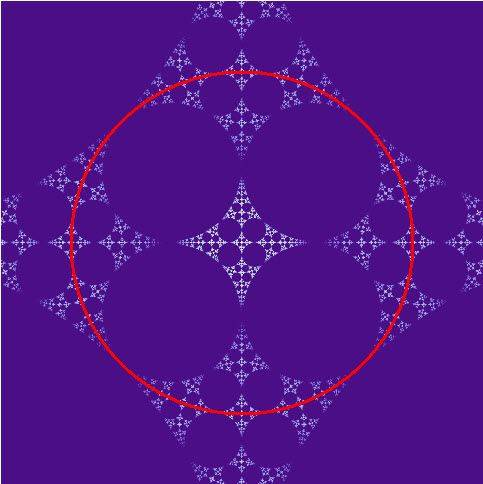The formula is:

$$R =\frac{1+k}{1-k}$$

For k=0.7 this gives R = 5.66...

It seems to work. But I do not know why it is so. And I do not know anything about the black holes.

Mentor
Interesting picture, and I have no idea how you generated it.

As far as I can see, all other diameters follow from geometry. The diameter of the big holes in the red circle satisfies (1+sqrt(2))*r = R, the smaller circles (similar to the big ones) have (1+sqrt(2))r' = (sqrt(2)-1)r.

$$r=\frac{R}{1+\sqrt{2}}$$
And we have a scaling factor of
$$s=\frac{\sqrt{2}-1}{\sqrt{2}+1} =(\sqrt{2}-1)^2$$

Interesting picture, and I have no idea how you generated it.

As far as I can see, all other diameters follow from geometry. The diameter of the big holes in the red circle satisfies (1+sqrt(2))*r = R, the smaller circles (similar to the big ones) have (1+sqrt(2))r' = (sqrt(2)-1)r.

$$r=\frac{R}{1+\sqrt{2}}$$
And we have a scaling factor of
$$s=\frac{\sqrt{2}-1}{\sqrt{2}+1} =(\sqrt{2}-1)^2$$

Wow! I did not notice it. And it was in plain sight. Thanks!

Explaining how the picture is generated would require some space. The same for my guess about the formula for the radius of the white circle (I calculated the infinitesimal area change of the square of the fourth transformation and looked for the solution of it being = 1).

Nope!

Nope. For k=0.6 we get this picture: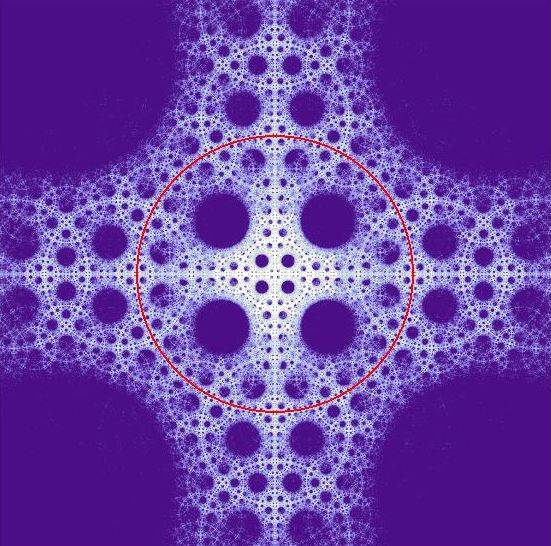Not that simple. My formula for the radius of the white circle still works - it is R=4 this time (The whole picture is from -8 to 8). But the smaller black circles do not touch. It was the particularity of k=0.7 that they seemed to be tangent.

Last edited:
•1 person
For k=0.6 we get this picture:

That's really neat!

That's really neat!

Thanks. Perhaps it is of interest that my six matrices describe six special Lorentz transformations, all with velocity v = 0.88 c, but in six different directions (+/- of each of three coordinate axes). The picture arises as the result of the relativistic light aberration when a monkey is piloting the UFO (able to instantly accelerate and change direction) with a joystick with six positions, and the monkey, looking at a fixed star, is randomly manipulating the joystick 100 mln times.

Update

I have found the algorithm for generating white (maximum density of hits) circles. The idea is that the Mobius group acts on the 3-dimensional space of circles (in mathematics this is a part of "Lie spheres geometry"). So given the transformations, and starting with one circle (say, a great circle on the sphere), we can generate generations of new circles. One can write the algorithm for doing this. There is no simple formula as we are dealing here with a composition of non-commuting maps (relativistic boosts in different directions) Here is the result (of course it makes no sense to generate circles that are too small to be seen as such on the image):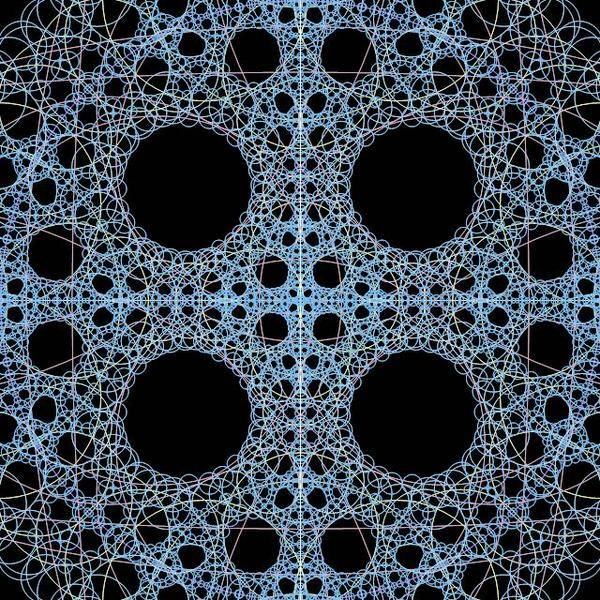They fit perfectly the structure that is seen on the fractal: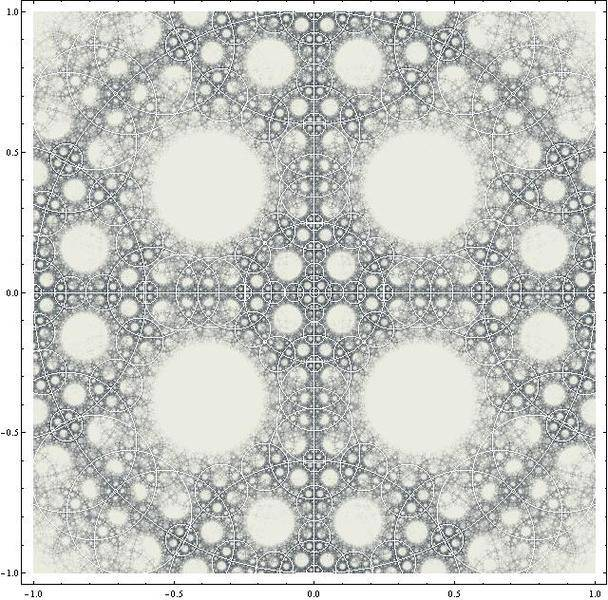(Colors inverted, circles and fractal overimposed).

With black circles it is more difficult. In fact thy are not circles - they are circle-like shapes contained in circles.